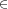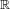## Chapter 12Notes for 3 September

Notes also available as PDF.

### 12.1 Proof review

2. A few tests, and the guess becomes a conjecture.
3. Once the conjecture is proven, you have a theorem.
• A lemma is a theorem leading to other theorems. Typically a technical result leading to the main theorem.
• A corollary is a subsequent theorem, a simple result after a primary theorem.

Other items:

• Definitions are difficult to define.
• Axioms are fundamental definitions. The classic example:

Two points define a line.

A direct proof takes the given hypotheses and applies rules directly to produce the conclusion. We used a direct proof to show that {n}^{2} is either a multiple of four (can be written as 4k for some k) or one larger than a multiple of four (4k + 1) when n is a non-negative integer.

An indirect proof , often called proof by contradiction or proof by contrapositive, proves that the opposite of the conclusion implies the opposite of the hypothesis. Thus if the hypothesis is true, the conclusion must be true. We used proof by contradiction to show that there are infinitely many primes. We actually proved that if there is a largest prime, then there is a larger number not divisible by any known prime and thus prime itself. The contradiction, constructing a prime larger than the largest prime, proved that there is no largest prime. Along with the known existence of primes, the fact there is no largest prime implies there must be infinitely many primes.

### 12.2 Inductive proof

Remember Pascal’s triangle? We proved that the sum of the nth row is {2}^{n} if we start counting rows from zero.

Written in rather boring table form, each entry is the sum of the entry directly above and above to the left:

 # 0 1 1 1 1 2 1 2 1 3 1 3 3 1 4 1 = \mathbf{0} + 1 4 = 1 + 3 6 = 3 + 3 4 = 3 + 1 1 = 1 + \mathbf{0}

We will use our result as an example of mathematical induction. This is a proof technique that formalizes inductive reasoning and using a representative case.

2. Then assume the nth case.
3. Use that to prove the (n + 1)st case.

We want to prove the following:

Theorem: The sum of the nth line of Pascal’s triangle is {2}^{n}.

Proof:

Here, the base case is the 0th line:

 # sum 0 1 1 = {2}^{0}

Then let line n be the sequence

 # sum n {P}_{n,1} {P}_{n,2} {P}_{n,3} \mathrel{⋯}\kern 1.54198pt {P}_{n,n+1} {2}^{n}

Now we need to prove that line (n + 1) holds true. In our notation, item jth on line (n + 1) is formed be {P}_{n+1,j} = {P}_{n,j-1} + {P}_{n,j}, setting the entries outside the table ({P}_{n,0} and {P}_{n,n+2}) to zero.

Then we need to show that {\mathop{\mathop{∑ }}\nolimits }_{j=1}^{n+2}{P}_{n+1,j} = {2}^{n+1}.

\eqalignno{ {\mathop{∑ }}_{j=1}^{n+2}{P}_{ n+1,j} & ={ \mathop{∑ }}_{j=1}^{n+2}\left ({P}_{ n,j-1} + {P}_{n,j}\right ) & & \cr & ={ \mathop{∑ }}_{j=1}^{n+2}{P}_{ n,j-1} +{ \mathop{∑ }}_{j=1}^{n+2}{P}_{ n,j} & & \cr & = \left ({P}_{n,0} +{ \mathop{∑ }}_{j=1}^{n+1}{P}_{ n,j}\right ) + \left ({P}_{n,n+2} +{ \mathop{∑ }}_{j=1}^{n+1}{P}_{ n,j}\right ) & & \cr & ={ \mathop{∑ }}_{j=1}^{n+1}{P}_{ n,j} +{ \mathop{∑ }}_{j=1}^{n+1}{P}_{ n,j} & & \cr & = {2}^{n} + {2}^{n} = 2 ⋅ {2}^{n} = {2}^{n+1}. & & }

### 12.3 Starting with set theory

Our coverage of set theory mostly will cover definitions. We will use some concepts to work with problem solving and to illustrate proofs. Then we will use sets to build up whole numbers (0, 1, 2, \mathop{\mathop{…}}) and basic arithmetic on those numbers.

### 12.4 Language of set theory

• We will cover just enough set theory to use later.
• Cardinalities are important for probability. We don’t have time to cover probability sufficiently well, so we will not explore the sizes of sets deeply.
• This is known as naïve set theory. We do not define absolutely everything, nor do we push set theory’s logical limits. Much.

Goals:

• Impart some of the language necessary for later chapters.
• Practice reasoning in a formal setting.
• One key aspect is what to do in extreme cases like empty sets.
• Set up straight-forward examples for logic.

### 12.5 Basic definitions

To start, we require unambiguous definitions of terms and items. When a term or item is unambiguously defined, it is called well-defined.

set
An unordered collection of unique elements.
• Curly braces: \{A,B,C\} is a set of three elements, A, B, and C.
• Order does not matter: \{\text{cat},\text{dog}\} is the same set as \{\text{dog},\text{cat}\}.
• Repeated elements do not matter: \{1,1,1\} is the same set as \{1\}.
• Can be implicit: \{x\kern 1.66702pt |\kern 1.66702pt x\text{ is an integer},x > 0,x < 3\} is the same set as \{1,2\}.
• Read the implicit form as “the set of elements x such that x is an integer, x > 0, and x < 3”. Or “the set of elements x where \mathop{\mathop{…}}
• Other symbols that sometimes stand for “such that”: :, (reversed)
• Implicit (or set-builder) form can include formula or other bits left of the bar. \{3x\kern 1.66702pt |\kern 1.66702pt x\text{ is a positive integer}\} is the set \{3,6,9,\mathop{\mathop{…}}\}.
element
Any item in a set, even other sets. (Also entry, member, item, etc.)
• This is not ambiguous. If something is in a set, it is an item of that set. It doesn’t matter if the item is a number or a grape.
• \{A,\{B,C\}\} is a set of two elements, A and \{B,C\}.
• None of the following are the same: \{A,\{B,C\}\}, \{A,B,C\}, \{\{A,B\},C\}.
empty set
Or null set. Denoted by rather than \{\}.
• This is a set on its own.
• \{∅\} is the set of the empty set, which is not empty.
• Think of sets as bags. An empty bag still is a bag, and if a bag contains an empty bag, the outer bag is not empty.
• Implicit definitions can hide empty sets.
• For example, the set \{x\kern 1.66702pt |\kern 1.66702pt x\text{ is an odd integer divisible by }2\} is .
singleton
A set with only one element.
• \{1\} and \{∅\} both are singletons (or sometimes singleton sets).

### 12.6 Translating sets into (and from) English

From English:

• The days of the week:
• \{\text{Monday},\text{Tuesday},\text{Wednesday},\text{Thursday},\text{Friday},\text{Saturday},\text{Sunday}\}
• Of course, we’re using a representation of the days and not the days themselves. That is how we reason about things; we model them and represent them by symbols.
• The days when homework is due:
• \{\text{25th of August},\text{1st of September},\mathop{\mathop{…}}\}
• We could list them all.
• \{ every Monday after the 18th of August 2008 until after the 1st of December \}
• Or: \{x\kern 1.66702pt |\kern 1.66702pt x is a Monday, x is after the 18th of August, and x is on or before the 1st of December \}

To English:

• \{2,3,4\}:
• The set containing two, three, and four.
• \{x\kern 1.66702pt |\kern 1.66702pt x\text{ is an integer and }x > 0\}:
• The positive integers, also called the counting numbers or the natural numbers.
• Often written as {J}^{+}. The integers often are written as J (because the “I” form can be difficult to read), rationals as (for quotients), the reals as.
• \{2x - 1\kern 1.66702pt |\kern 1.66702pt x{J}^{+}\}
• The set whose members have the form 2x - 1 where x is a positive integer.
• Cannot list all the entries; this is an infinite set.
• Here, the odd integers.

### 12.8 Homework

Groups are fine, turn in your own work. Homework is due in or before class on Mondays.

Write out (briefly) your approach to each problem.

• Prove the formula for the sum of the first n positive integers by mathematical induction. That is, prove 1 + 2 + 3 + \mathrel{⋯} + n = n(n + 1)∕2. The base case here is n = 1. Then show that the nth term transforms into the (n + 1)th term by adding n + 1.
• Problem set 2.1 (p83):
• Problems 1, 2, 4, 5, 6, 27

Note that you may email homework. However, I don’t use MicrosoftTM products (e.g. Word), and software packages are notoriously finicky about translating mathematics.

If you’re typing it (which I advise just for practice in whatever tools you use), you likely want to turn in a printout. If you do want to email your submission, please produce a PDF or PostScript document.